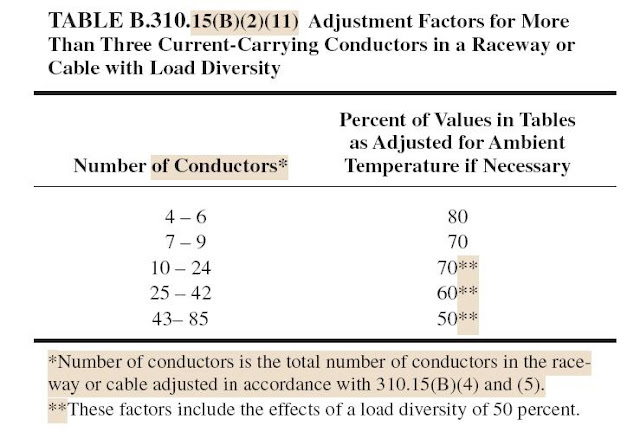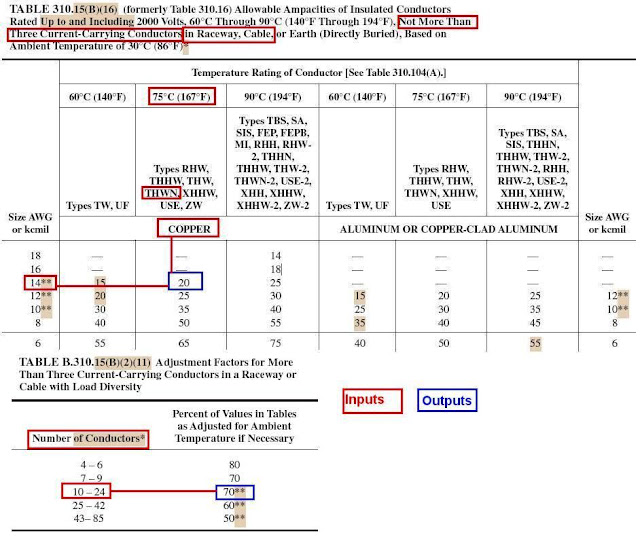### Conductor Ampacity Calculation – Part Twelve

In Article " Conductor Ampacity Calculation – Part Eleven ", I explained how to use the Annex B Tables included under Group#2-B “tables for conductors directly Buried in earth” which includes:

• TABLE B.310.15(B)(2)(8) in Accordance with Figure B.310.15(B)(2)(2),
• TABLE B.310.15(B)(2)(9) in Accordance with Figure B.310.15(B)(2)(2),
• TABLE B.310.15(B)(2)(10) in Accordance with Figure B.310.15(B)(2)(2),

Also,In Article " Conductor Ampacity Calculation – Part Ten ", I explained how to use the Annex B Tables included under Group#2-A “ tables for conductors installed in underground electrical duct bank” which includes:

• TABLE B.310.15(B)(2)(5) in Accordance with Figure B.310.15(B)(2)(2),
• TABLE B.310.15(B)(2)(6) in Accordance with Figure B.310.15(B)(2)(2),
• TABLE B.310.15(B)(2)(7) in Accordance with Figure B.310.15(B)(2)(2),

Today, I will explain how to use Other Annex B tables and figures.

For more information about the Second method for Conductors ampacity calculations by using Annex B Tables under engineering supervision, please review the following articles:

For more information about the first method for Conductors ampacity calculations by using Tables as provided in 310.15(B), please review the following articles:

Using Annex B tables and figures:

 Rule#1: Annex B Tables And Figures Annex B consists of many tables and figures as follows: 1- Tables: TABLE B.310.15(B)(2)(1), TABLE B.310.15(B)(2)(3), TABLE B.310.15(B)(2)(5) in Accordance with Figure B.310.15(B)(2)(2), TABLE B.310.15(B)(2)(6) in Accordance with Figure B.310.15(B)(2)(2), TABLE B.310.15(B)(2)(7) in Accordance with Figure B.310.15(B)(2)(2), TABLE B.310.15(B)(2)(8)  in Accordance with Figure B.310.15(B)(2)(2), TABLE B.310.15(B)(2)(9) in Accordance with Figure B.310.15(B)(2)(2), TABLE B.310.15(B)(2)(10) in Accordance with Figure B.310.15(B)(2)(2), TABLE B.310.15(B)(2)(11). 2- Figures: The following figures represent Underground electrical duct bank configurations: Figure B.310.15(B)(2)(1) Figure B.310.15(B)(2)(2) Figure B.310.15(B)(2)(3), Figure B.310.15(B)(2)(4), Figure B.310.15(B)(2)(5).

Annex B tables and figures, click on the link.

The above tables can be divided to groups as follows:

• Tables Group#1: tables for conductors installed in free air.
• Tables Group#2: tables for conductors installed in underground electrical duct bank tables.
• Tables Group#3: adjustment factor tables

The above figures can be divided to groups as follows

• Figures Group#2-A: Detail Figures.
• Figures Group#2-B: special case figures.

Third: Tables Group#3: adjustment factor tables

This tables group includes One Table only which is TABLE B.310.15(B)(2)(11).

1.1 Tables Group#3 Construction:Table B.310.15(B)(2)(11) consists of: (see above image)

 1.1.A Conditions For Table Application These conditions are existing in the table description at the top of the table, and in table  B.310.15(B)(2)(11) , these conditions are: More Than Three Current-Carrying Conductors, in a Raceway or Cable, With Load Diversity.

 1.1.B Conductor Material Sections Two main Columns for conductor material type are existing: Number of Conductors (from 4 to 85 conductors), Percent of Values in Tables as Adjusted for Ambient Temperature if Necessary (ranges from 50 to 80%).

1.2 Notes for Tables Group#3:

 Note#1 The Application  Conditions for table B.310.15(B)(2)(11) limit the use of it to the following tables: Table 310.15(B)(16), Table 310.15(B) (18), Table B.310.15(B)(2)(1), Table B.310.15(B)(2) (6), Table B.310.15(B)(2)(7)

 Note#2 The asterisk (*) next to Number of conductors means that: The Number of conductors is the total number of conductors in the raceway or cable adjusted in accordance with 310.15(B)(4) and (5). For more information about using Rules of 310.15(B)(4) and (5), please review our Article " Conductor Ampacity Calculation – Part Six ".

 310.15(B)(4): Bare or Covered Conductors Where bare or covered conductors are installed with insulated conductors, the temperature rating of the bare or covered conductor shall be equal to the lowest temperature rating of the insulated conductors for the purpose of determining ampacity.

 310.15(B)(4): Neutral Conductor This section discuss considering the neutral conductor as a current carrying conductor or not. If it will be a current carrying conductor it will be counted when applying the Adjustment factor of table B.310.15(B)(2)(11) where is More Than Three Current-Carrying Conductors in a Raceway or Cable.

 Definition: Nonlinear Load:  A load where the wave shape of the steady-state current does not follow the wave shape of the applied voltage.

 Note#3 The asterisk (**) means that These adjustment factors in table B.310.15(B)(2)(11) for Number of conductors from 10 to 85,  include the effects of a load diversity of 50 percent.

 Note#4 The ampacity limit for 10 through 85 current-carrying conductors is based on the following equation: where: A1 = ampacity from Table 310.15(B)(16), Table 310.15(B) (18), Table B.310.15(B)(2)(1), Table B.310.15(B)(2) (6), or Table B.310.15(B)(2)(7) multiplied by the appropriate adjustment factor from Table B.310.15(B) (2)(11). N = total number of conductors used to select adjustment factor from Table B.310.15(B)(2)(11), E = number of conductors carrying current simultaneously in the raceway or cable, A2 = ampacity limit for the current-carrying conductors in the raceway or cable For more than 85 conductors, special calculations are required that are beyond the scope of this table.

1.3 How to use Tables group#3 (Table B.310.15(B)(2)(11))

Example# 1:

Calculate the ampacity limit for twelve 14 AWG THWN current-carrying conductors (75°C) in a raceway that contains 24 conductors that may, at different times, be current carrying.

Solution:

Step#1: determine tha ampacity for 14 AWG, THWN, Copper conductors (75°C) in a raceway.

Use table Table 310.15(B)(16), you get ampacity reading = 20 AStep#2: apply the above equation to get the adjusted ampacity , given the following data:

A1 = ampacity from Table 310.15(B)(16) from Step#1 = 20 A

N = total number of conductors = 24

E = number of conductors carrying current simultaneously in the raceway or cable = 12

SO,Example# 2:

Calculate the ampacity limit for eighteen 14 AWG THWN current-carrying conductors (75°C) in a raceway that contains 24 conductors that may, at different times, be current-carrying.

Solution:

Step#1: as in Example#1 above.

Step#2: apply the above equation to get the adjusted ampacity , given the following data:

A1 = ampacity from Table 310.15(B)(16) from Step#1 = 20 A

N = total number of conductors = 24

E = number of conductors carrying current simultaneously in the raceway or cable = 18

SO,This figures group include one figure only which is Figure B.310.15(B)(2)(1).

Using Figure B.310.15(B)(2)(1)

All three tables included in group#2-A have same values of thermal resistivity (RHO) and Load Factor (LF) which are as follows:

 RHO RHO RHO 60 90 120 LF LF LF 50 100 100

But if in some installations we have different values of thermal resistivity (RHO) and Load Factor (LF), what will be done?

The answer is using Figure B.310.15(B)(2)(1) in below image, which make Interpolation for the values of current ampacity for these installations based on two known ampacities for standard values of thermal resistivity (RHO) and Load Factor (LF).

 Tables Group#2A This tables group includes the following tables:  TABLE B.310.15(B)(2)(5) in Accordance with Figure B.310.15(B)(2)(2), TABLE B.310.15(B)(2)(6) in Accordance with Figure B.310.15(B)(2)(2), TABLE B.310.15(B)(2)(7) in Accordance with Figure B.310.15(B)(2)(2),

Fifth: Figures Group#2-A: Detail Figures

This figures group include one figure only which is Figure B.310.15(B)(2)(2).

 Using Figure B.310.15(B)(2)(2) All tables included under group#2 are used in Accordance with electrical duct bank configurations included in Figure B.310.15(B)(2)(2). (see below table)

 Tables Group#2: tables for conductors installed in underground electrical duct bank or directly Buried tables This tables group can be divided to two sub-groups as follows: 1- Tables Group#2-A: tables for conductors installed in underground electrical duct bank which includes: TABLE B.310.15(B)(2)(5) in Accordance with Figure B.310.15(B)(2)(2), TABLE B.310.15(B)(2)(6) in Accordance with Figure B.310.15(B)(2)(2), TABLE B.310.15(B)(2)(7) in Accordance with Figure B.310.15(B)(2)(2), 2- Tables Sup-Group#2-B: tables for conductors directly Buried in earth which includes: TABLE B.310.15(B)(2)(8)  in Accordance with Figure B.310.15(B)(2)(2), TABLE B.310.15(B)(2)(9) in Accordance with Figure B.310.15(B)(2)(2), TABLE B.310.15(B)(2)(10) in Accordance with Figure B.310.15(B)(2)(2),

 Table B.310.15(B)(2)(5) Table B.310.15(B)(2)(6) Table B.310.15(B)(2)(7) Allowable Electrical Duct Configurations from Figure B.310.15(B)(2)(2) Detail 2 ,3 and 4 Detail 1 ,2 and 3 Detail 1 ,2 and 3
 Table B.310.15(B)(2)(8) Table B.310.15(B)(2)(9) Table B.310.15(B)(2)(10) Allowable Cable Installation Configurations from Figure B.310.15(B)(2)(2) Detail 5 and 6 Detail 7 and 8 Detail 9 and 10

 Note#1 Minimum burial depths to top electrical ducts or cables shall be in accordance with 300.5. Maximum depth to the top of electrical duct banks shall be 750 mm (30 in.) and maximum depth to the top of direct buried cables shall be 900 mm (36 in.)

 Note#2 For two and four electrical duct installations with electrical ducts installed in a single row, see B.310.15(B)(5).

In the next Article, I will explain how to use the last figures group#3 included in NEC Annex B. Please, keep following.